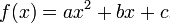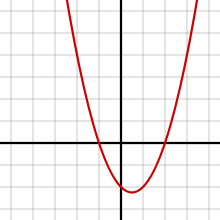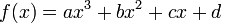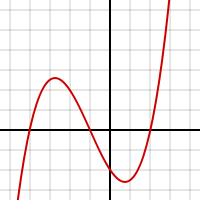A quadratic equation (or function) is one that has this form:Think of f(x) = y if you feel more comfortable with that. In other words, y is a function of both x and x-squared. This is called a polynomial of degree two or a second order polynomial. Quadratic equations define parabolas.A cubic equation (or function) is one that has this form:Again, think of f(x) = y if you feel more comfortable with that. In other words, y is a function of x, x-squared, and x-cubed. This is called a polynomial of degree three, or a third order polynomial. Cubic equations define curves of the following general form.Once again, to keep my math friends happy and be completely technically correct, we would have to replace f(x) or y with 0 for these to be "equations," but in statistics we call them equations. Return To Main Page